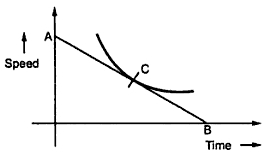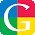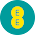Retardation Test or Running Down Test

This method is generally employed to shunt generators and shunt motors. From this method we can get stary losses. Thus if armature and shunt copper losses at any given load current are known then efficiency of a machine can be easily estimated.
The connections required for conducting this test are shown in the Fig. 1.
The machine whose test is to be taken is run at a speed which is slightly above its normal speed. The supply to the motor is cut off while the field is kept excited. The armature consequently slows down and its kinetic energy is used in supplying the rotational or stray losses which includes iron, friction and winding loss.
If I is the amount of inertia of the armature ans is the angular velocity.
Kinetic energy of armature = 0.5 Iω2
...   Rotational losses, W = Rate of change of kinetic energyFig. 1 Retardation test
Angular velocity, ω = (2 πN)/60

Thus if we if to find the rotational losses, the moment of inertia I and dN/dt must be known.
These quantities can be found as follows ;-
1.1 Determination of dN/dt
The voltmeter  V1 which is connected across the armature will read the back e.m.f. of the motor. We know that back e.m.f. is proportional to speed so that voltmeter is calibrated to read the speed directly.
When motor is cut off from the supply, the speed decrease in speed is noted with the help of stop watch. A curve showing variation between time and speed which is obtained from voltmeter which is suitably calibrated is shown in the Fig. 2.Fig. 2
At any point C corresponding to normal speed, a tangent AB is drawn. Then
The value obtained from above can be substituted in the expression for W which can give the rotational looses.
1.2 Determination of moment of inertia (I)
Method (a) Using Flywheel
The armature supply is cut off and time required for definite change in speed is noted to draw the corresponding curve as we have drawn in previous case. This curve is drawn considering only armature of the machine. Now a flywheel with known moment of the inertia say is  I1 keyed onto the shaft and the same curve is drawn again. The slowing down time will be extended as combined moment of inertia of the two is increased.
For any given speed (dN/dt1) and (dN/dt2) are determined same as previous case. It can be seen that the losses in both the cases are almost same as addition of flywheel will not make much difference to the losses.
In the first case where flywheel is not there then,
Adding the flywheel to the motor armature in second case we get,
Method (b) Without using Flywheel
In this method time is noted for the machine to slow down by say 5 % considering the armature alone. The a retarding torque either mechanical or electrical is applied. Preferably electrical retarding torque is applied and time required to slow down by 5% is noted again. The method by which electrical torque can be provided is shown in the Fig. 1 in which the switch S after disconnecting from the supply is thrown to terminals 1'2'. The machine then gets connected to a non-inductive load resistance RL. The power drawn by this resistance will acts as a retarding torque on the armature which will make it slow more quickly.
The additional loss in the resistance will be equal to product of ammeter reading and the average reading of the voltmeter (for a fall of 5% of voltmeter reading, the time is noted.) The ammeter reading is also changing so its average reading is taken. Thus the additional losses is Ia2 (Ra + R). Let t1 be the time when armature is considered alone and t2 be the time when armature is connected across a load resistance, V be average voltage across R and Ia be the average current and W' is additional retarding electrical torque supplied by motor.
If dN i.e. change in speed is same in two cases then

Here dN/dt1 is rate of change in speed without extra load whereas dN/dt2 is rate change in speed with extra electrical load which provides retarding torque.
Example :
A retardation test is made on a separately excited d.c. machine as a motor. The induced voltage falls from 240 V to 220 V in 25 seconds on opening the armature circuit and in 6 seconds on suddenly changing the armature connection from supply to a load resistance which takes average current of 10 A. Find the efficiency of the machine when running as a motor taking a current of 25 A on a supply of 250 V. The resistance of its armature is 0.3 Ω and that of its field winding is 200 Ω.
Solution :
Iav = 10 A
Average voltage across load = (240+220) / 2 = 230
Power absorbed,   W' = Vav Iav = 230 x 10 = 2300 W
t1 = 25 sec
t2 = 6 sec
Stary losses = 726.31 W
Motor in input current, IL = 25 A
Shunt current, Ish = V/Rsh = 250/200 = 1.25A
Armature current, Ia = IL - Ish = 25-1.25 = 23.75 A
Armature copper loss = Ia2 Ra = (23.75)2 x 0.3 = 169.21 W
Shunt copper loss = Ish2 Rsh = (1.25)2 x 200 = 312.5 W
Total losses = 169.21 + 312.5 + 726.31 = 1208.02 W
Input to the motor = V .I = 250 x 25 = 6250 W
Output of motor = Input - Losses = 6250 - 1208.02 = 5041.98 W
%Efficiency of motor ηm = (Output/Input) x 100 = (5041.98/6250) x 100 = 80.67
η = 80.67 %

Related Articles :
Torque Equation of a D.c. Motor
Characteristics of D.C. Shunt Motor
Necessity of Starter for D.C. Motors
Brake Test

1.2.3.